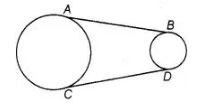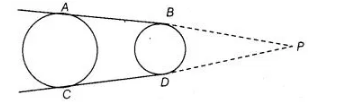# In figure, AB and CD are common tangents

Question:

In figure, AB and CD are common tangents to two circles of unequal radii. Prove that AB = CDSolution:

Given AS and CD are common tangent to two circles of unequal radius

To prove                                        AB = CDConstruction Produce $A B$ and $C D$, to intersect at $P$.

Proof $P A=P C$

[the length of tangents drawn from an internal point to a circle are equal]

Also, $P B=P D$

[the lengths of tangents drawn from an internal point to a circle are equal]

$\therefore$ $P A-P B=P C-P D$

$A B=C D$

Hence proved.